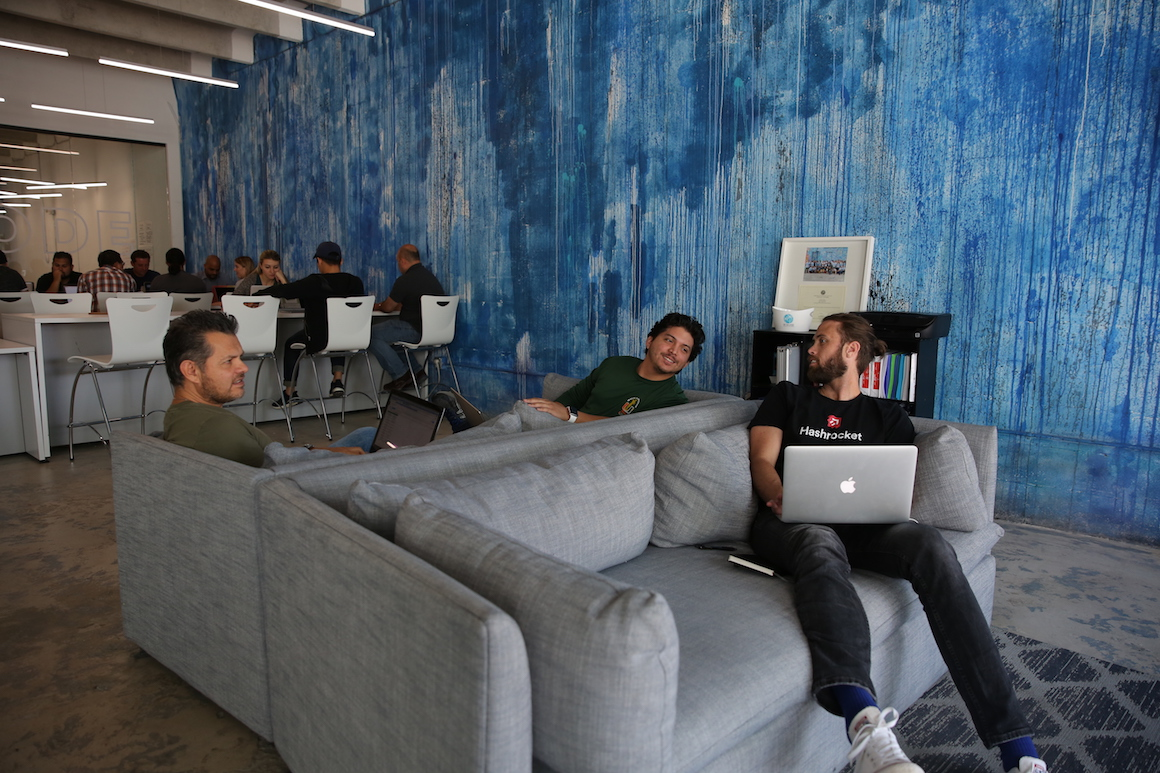Want to gain a deeper understanding of big-o notation, recursion, boolean algebra, set theory, number theory, graph theory, probability, statistics & how to formulate a proof to back up the correctness of your algorithm?Wyncode is hosting a 12 weekend long study group to cover videos on all of these topics in depth. We will be reviewing video content covering the following topics:

1. Application of boolean algebra
2. Introduction to set theory
3. Formulating proofs
4. Understanding and formulating proofs by Induction
5. Understanding and formulating proofs by Strong Induction
6. Number theory with state machines
7. Introduction to encryption via number theory
8. Graph theory and it's applications
9. Graph algorithms (such as minimum spanning trees, topological sorts and more)
10. Introduction to matching problems
11. Introduction to asymptotic (Big-O) notation
12. Introduction to recurrence relations (linear as well as "divide and conquer")
13. Defining asymptotes for recurrences
14. Vocabulary for statistics
15. Basics of probability
16. Introduction to conditional probability
17. Understanding dependence or independence of variables
18. Basics of linearity of expectation / expected value

Led by Tim Reen (Bachelors in Mathematics) and Auston Bunsen, participants will be able to stop the video at any moment to ask for clarification on a particular topic or item of interest.

This group will meet every Saturday from 10am to approx. 2:30pm, the schedule will be as follows:

10:00AM Start screening of video on topic

11:30AM Split into 2 groups and work on problem set

12:00PM Meet as class to address any questions

12:15-1:00PM Lunch break (lunch not provided)

1:00PM Start screening of video on next topic

2:20PM Split into 2 groups and work on next problem set

People that attend this group should have at least a high school level of math, including Algebra & Geometry. Statistics & Calculus experience is a plus!